# Given zeo fuel mass 4920 trip fuel

Classified in Chemistry

Written at onEnglish with a size of 32.08 KB.

Combustion is a major source of air pollution emissions. The complete combustion of hydrocarbon fuel produces only CO2 and H2O as products, according to the following stoichiometry:

CH4 + 2 O2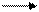CO2 + 2 H2O

a) Mass of air required for complete combustion of 500 kg of CH4, assuming O2 = 21% of air.

b) Mass of CO2 produced from the above combustion

c) Calculate the mass of air required for combustion of 500 kg of benzene (C6H6).

a) Mass of O2 required =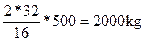Mass of air required= 2000/0.23=8696  kg

b) Mass of CO2 produced=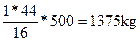c)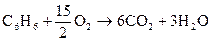Mass of oxygen required=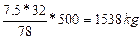Mass of air required= 1538/0.23= 6686 kg

Mass of CO2 produced = (6 x 44)/78 x 500 = 1692 kg

Fuel oil contains the following components (by weight); C- 88.3%, H- 9.5%, S- 1.6%, ash- 0.1%

1. Calculate the stoichiometric air required if 1 kg fuel oil is combusted completely.
2. Determine the SO2 emission from this process.

For C, O2 required =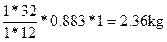Air required = 2.36/0.23 = 11.24 kg

For H,O2 required =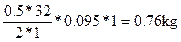Air required = 0.76/0.23 = 3.30 kg

For S,O2 required =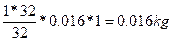Air required = 0.016/0.23= 0.069 kg

Total amount of air required = 10.26+3.30+0.069 = 13.6 kg

SO2 emitted = (64/32)*0.016*1 = 0.032 kg

Industrial representatives claim that the NAAQS for SO2 is so low that one exceeds it if one strikes a simple wooden match in a modest sized room. Is this true?

• Calculate the concentration expected for striking such a match in a room that is 15 ft by 15ft by 8ft. A typical 2-inch wooden match contains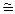2.5 mg of sulfur.
• Compare the resulting concentration to the annual average SO2 NAAQS standard. The NAAQS for SO2 (annual average) is 80 mg/m3.

S + O2® SO2

Mass of SO2 = 64 * 2.5 = 5 mg

32

Conc. Of SO2 =         5 mg          *            ft3

(15 * 15 * 8) ft3        0.028 m3

= 0.099 mg/m3

= 99 mg/m3 > 80 mg/m3

So the conc. Of SO2 will exceed the NAAQS standard.

Calculate the total emission rate for CO in kg/yr, assuming CEMS data for CO as 1600 hr/yr for the period and has an exhaust gas temperature of 180°C. The CEM output for concentration of CO is 54.9 (ppmvd) from a furnace firing waste fuel oil and the oxygen content is 10.5 % by volume, gas flow rate is 8.66 m3/s and the production rate of product is 276 ton/hr.

For CO®M = 12 + 16 = 28

P = 1 atm,    T = 180°C = 453K,   Q = 8.66 m3/s,   R = 0.0821 L.Atm/mol. °K

ECO            = Y* P * M  * Q

R * T

=54.9 x 10-6 mol/mol * 1 atm x 28 g/mol * 8.66 m3/s

0.0821 L. Atm/mol.°K * 453°K * m3/1000L

= 0.358 g/s * kg/1000 g * 3600 sec/hr = 1.29 kg/hr

• ECO total = 1.29 x 1600 = 2064 kg/yr

Estimate the emission rate of particulate matter (PM10) if 2.5 kg of PM10 is emitted from a steam tube dryer in a canning and by-product manufacturing facility, for each ton of raw fish processed. The overall control efficiency of the pollutant is 40%. It is assumed that the facility operates for 10 hrs/d and 260 days/year, and the activity rate is 5 ton/hr.

Emission Factor = 2.5 kg/ton

Efficiency= 40%=0.4

Operating hour=10*260=2600hr/year

Activity rate= 5 ton/hr

Emission rate=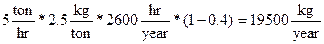Calculate the mole fraction of H2S in water that is in contact with air at 20°C and contains H2S with partial pressure of 0.05 atm.

kH of H2S at 20°C is 4.83 x 102 atm/mol fraction.

0.05 = 4.38*102*C

C=1.1*10-4 mol fraction

Typical person at rest produces 0.007 ml/min of CO and the typical human diet in wealthy countries like Canada is about 600 g/day of food (dry basis). Calculate the emission factor for CO by human (g CO/g food).

What is the emission factor for automobiles, in lb CO/ton of fuel burned? The permitted CO emission for new cars is 3.4 g/mile. Assume that the fuel economy is 25 mile/gal and the gasoline density is 0.72 kg/L.Emission factor for CO

A gas kitchen stove consumes 3000 kcal/h of fuel. The emission factor for NO­2 for that kind of stove is about 61 µg/kcal. The air infiltration rate is 3000 ft3/h and the outdoor concentration 20 µg/m3; there is no destruction of NO2; estimate the steady state concentration when the stove has run long enough to reach steady sate.

Emission rate = E =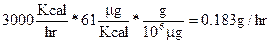Q = 3000 ft3/h = 84.95 m3/hr, k=0

Ca = 20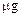/ m3

C =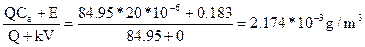C = 2174/m3

A bar with volume 500 m3 has 50 smokers in it, each smoking two cigarettes per hour. An individual cigarette emits, among other things, about 1.4 mg of formaldehyde (HCHO). Formaldehyde converts to carbon dioxide with reaction rate coefficient k = 0.40 h-1. Fresh air enters the bar at the rate of 1000 m3/hr, and stale air leaves at the same rate. Assuming complete mixing,

a) Estimate the steady state concentration of formaldehyde in the air at 25°C and 1 atm of pressure.

b) How does the result compare with the threshold for eye irritation of about 0.05 ppm?

k=0.4 h-1, V = 500 m3, Q=1000 m3/hr, Ca = 0.0

MWHCHO = 30 g/mol

Emission rate = E = 50*2*1.4 = 140 mg/hr

C=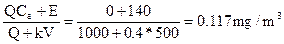C (mg/m3) = Cppm* PM/RT

0.117 = Cppm * (1*30) / (0.08206*298)

Cppm = 0.095 ppm

The sidestream smoke from one cigarette releases about 0.1mg of benzo(a) pyrene (BaP). In an apartment, with fresh air entering through holes and cracks (infiltration) at an average rate of 120 m3/hr. The potency factor inhalation route for benzo(a) pyrene is 6.11 (mg/kg-d)-1 as per USEPA.

• What would be the steady-state indoor concentration of BaP if one cigarette per hour is smoked? (Assume that BaP is a conservative pollutant).
• What would be the incremental cancer risk to a non-smoking roommate who spends 8 hr/day for 1 year in this apartment? (Assume an inhalation rate of 20m3/day).
• Input rate = output rate

1 cig/hr x 0.1 mg/cig = 120m3/hr x C mg/m3

C = 0.1/120 = 0.00083 mg/m3

b) Living for a year with a smoker:

Chronic Daily Intake, (CDI)   = 0.00083 mg/m3 x20m3/day x 1 day/24hr x 8 hr/day x 365 day

70 kg x 365 d/yr x 70 yr

= 1.1 x 10-6 mg/kg-d

R = 1.1 x 10-6 mg/kg-d x 6.11 (mg/kg-d)-1

= 7 x 10-6

A Container of Carbon Tetrachloride (CCl4) is left open in an unventilated cabinet. An individual opens the doors and is exposed to it. What is the carbon tetrachloride concentration in ppm that the employee is exposed to? (The vapour pressure of (CCl4) at 20°C is 0.12atm).

Solution:

Vapour pressure of CCl4 = 0.12 atm

P1 V1 = n1 R T1    for CCl4

P2 V2 = n2 R T2   for air

P1 = 0.12 atm             P2 = 1 atm

V1 = V2         T1 = T2

n1/n2 = P1 /P2

Concentration of CCl4 = n1/n2 = P1 /P2

= (0.12 atm / 1atm) x 106

= 120000 ppm

Therefore the employee will be exposed to 120000 ppm of CCl4

Ethyl alcohol (C2H5OH) is accidentally spilled on the floor of a store room in which the temperature is 23°C, 1 atm.   The average air velocity is 2 m/s and the spill is 2 m in diameter.  Estimate the rate of evaporation in kg/hr.

Calculate the vapor composition of 50 mol% benzene and 50 mol% toluene in equilibrium with air in a closed container.  Vapor pressures of benzene and toluene are 1.45 psi and 0.42 psi, respectively.

(b)Aqueous solution of toluene with mole fraction xtol= 9.77 x 10-5; Toluene vapor pressure @ 25 0C =29.8 mm Hg; Henry’s law constant = 3.72 x 107 N/m2.  Calculate the partial pressure of toluene over the aqueous solution at 25 0C using Raoult’s law and Henry’s Law.ybenzene = xbenzene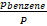= 0.5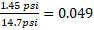ytoulene = xtoluene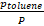= 0.5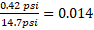yair = 1-0.049-0.014= 0.937

Pi= xi H= (3.72 x 107 N/m2) (9.77 x 10-5) = 3634 N/m2

Pj = xj Pv,j= (9.77 x 10-5) ( 29.8/760 atm) (105 Nm-2 atm -1) = 0.382 N/m2